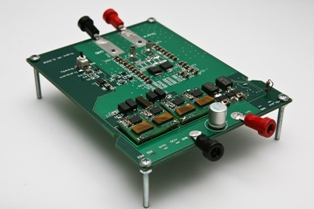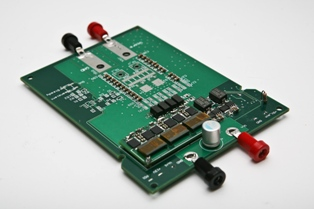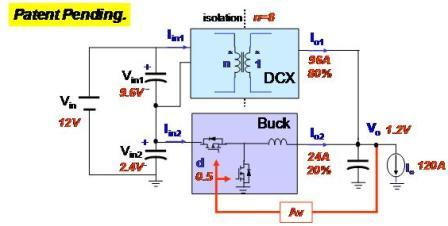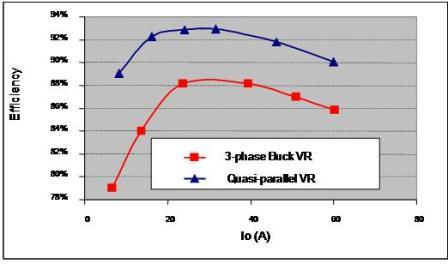LIBRARY

# High efficiency Sigma VRs (2007)

Year: 2007High efficiency is generally required for CPU VR. For example, 90% plus efficiency is desired for laptop VR to extend the battery life. High efficiency is also expected for server VR to save energy and electricity bills since there are many microprocessors in server systems, especially in server farm. It is very hard for current multi-phase Buck VR to meet future efficiency requirement.

To achieve high efficiency with low cost, the sigma VR structure is proposed, as shown in Fig. 1. It composes Buck converter and high efficiency DC/DC transformers. The inputs of two converters are in series, while the outputs are in parallel. The power ratio is equal to the input voltage ratio for the two converters. As a result, the power ratio can be controlled by n. For example, if n=8, Vo = 1.2V, then Vin1 = 9.6V, Vin2=2.4V, Vin1/Vin2 = 4:1. That means high efficiency DCX handles 80% output power, while Buck only needs to provide 20% output power.

By taking advantage of CPES inductor-less topology, more than 90% efficiency can be achieved by DC/DC transformer. Since the sigma converter efficiency is dominated by DCX efficiency, it can achieve around 90% efficiency at 600kHz frequency, which is 4% higher than state-of-the-art multi-phase Buck VR for server applications.Fig. 1. Sigma VR structure.Fig. 2. Efficiency comparison between sigma VR and multi-phase Buck VR (600kHz).